Courses

# Water Requirements of Crops Civil Engineering (CE) Notes | EduRev

## Civil Engineering (CE) : Water Requirements of Crops Civil Engineering (CE) Notes | EduRev

The document Water Requirements of Crops Civil Engineering (CE) Notes | EduRev is a part of the Civil Engineering (CE) Course Irrigation Engineering.
All you need of Civil Engineering (CE) at this link: Civil Engineering (CE)

Q. 1 The command area of a canal grows only one crop, i.e., wheat. The base period of wheat is 120 days and its total water requirement, A, is 40 cm. If the canal discharge is 2 m3/s, the area, in hectares, rounded off to the nearest integer, which could be irrigated (neglecting all losses) is______   [2019 : 1 Mark, Set-II]
Ans: 5184 ha

Given data:
Base period,
B = 120 days
Delta of crop,
Δ = 40 cm
Discharge, Q= 2 m3/s
Area to be irrigated,
A = ?

∴ Duty of water,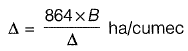and Area to be irrigated;
A = Q x D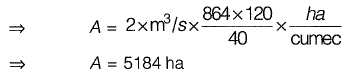Q. 2 The intensity of irrigation for the Kharif season is 50% for an irrigation project with culturable command area of 50,000 hectares. The duty for the Kharif season is 1000 hectare cumec. Assuming transmission loss of 10%, the required discharge (in cumec, up to two decimal places) at the head of the canal is __________ . [2018 : 1 Mark, Set-II]
Ans: 27.78cumec
Culturable command area = 50000 ha
Intensity of irrigation for kharif season = 50%
∴Area under kharif = 25000 ha
Duty for kharif season = 1000 ha/cumec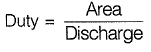∴ Discharge at the head of field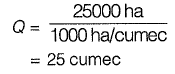Transmission/conveyance loss = 10%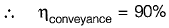Discharge at the head of canal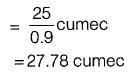Q. 3 The culturable command area of a canal is 10000 ha. The area grows only two crops-rice in the Kharif season and wheat in the Rabi season. The design discharge of the canal is based on the rice requirements, which has an irrigated area of 2500 ha, base period of 150 days and delta of 130 cm. The maximum permissible irrigated area (in ha) for wheat, with a base period of 120 days and delta of 50 cm, i s _________ . [2017 : 2 Marks, Set-II]
Ans: 5199.966 ha ≈ 5200 ha

Method I
CCA = 10000 ha
For rice, Δr = 130 cm = 1.3 m
Ar = 2500ha
Br = 150 days

Duty,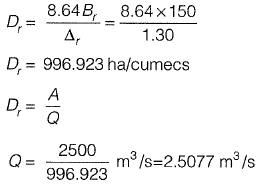For wheat also, discharge is 2.5077 m3/s,
Bw = 120days
Δ = 50 cm
Aw  = Qx Dw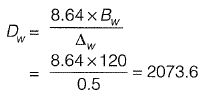∴ Aw = 2.5077 x 2073.6
= 5199.966 ha ≈ 5200 ha

Method II
Dischage,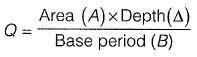Now, equate Q for ricve and wheat
Hence, Qr = Qw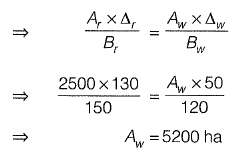Q. 4 Field channel has cultivable commanded area of 2000 hectares. The intensities of irrigation for gram and wheat are 30% and 50% respectively. Gram has a kor period of 18 days, kor depth of 12 cm, while wheat has a kor period of 18 days and a kor depth of 15 cm. The discharge (in m3/s) required in the field channel to supply water to the commanded area during the kor period is ___________.   [2015 : 2 Marks, Set-II]
Ans: 1.4275 m3/s

Gram and wheat are Rabi crops.

So both are grown in the same period.

Discharge required for gram,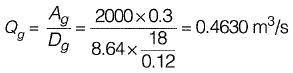Discharge required for wheat,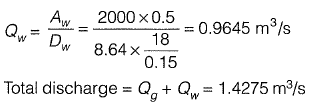Q. 5 The two columns below show some parameters and their possible values.

Parameter:
P. Gross Command Area
Q. Permanent Wilting Point
R. Duty of canal water
S. Delta of wheat
Value:
I. 100 hectares/cumec
II. 6°C
III. 1000 hectares
IV. 1000 cm
V. 40 cm
VI. 0.12

Which of the following options matches the parameters and the values correctly?
(a) P-I, Q-ll, R-lll, S-IV
(b) P-lll, Q-VI, R-I, S-V
(c) P-I, Q-V, R-VI, S-ll
(d) P-lll, Q-ll, R-V, S-IV
[2015 : 1 Mark, Set-I]
Ans: (B)

Gross Command Area is measured in hectares. (P-III)

Duty has a unit of ha/cumec. (R-I)
Delta of wheat can be practically 40 cm ( S - V )

Q. 6 Irrigation water is to be provided to a crop in a field to bring the moisture content of the soil from the existing 18% to the field capacity of the soil at 28%. The effective root zone of the crop is 70 cm. It the densities of the soil and water are 1.3 g/cm3 and 1.0 g/cm3 respectively, the depth of irrigation water (in mm) required for irrigating the crop i s ________ .    [2014 : 2 Marks, Set-II]
Ans: 91mm
Given,
Root zone depth,
d = 70 cm
Field capacity,
Fc = 28%
Existing moisture content,
w = 18%
Density of soil,
γ = 1.3 gm/cm3
Density of water,
γw = 1.0 gm/cm3
Depth of irrigation water required,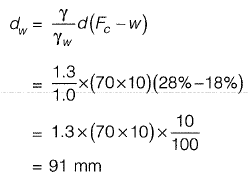Note: It is to be understood that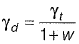,  but as it is not mentioned clearly in the question that the density of 1.3 g/cc is computed at which moisture content, hence, assuming the given density of 1.3 g/cc to be the dry density.

If it would have been given that density of 1.3 g/cc is at moisture content 18%, then we would have computed the dry density from the formula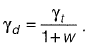Q. 7  The transplantation of rice requires 10 days and total depth of water required during transplantation is 48 cm. During trans-plantation there is an effective rainfall (useful for irrigation) of 8 cm. The duty of irrigation water in hectare/cumecs is
(a) 612
(b) 216
(c) 300
(d) 108    [2013 : 2 Marks]
Ans: (b)
Δ = Total water depth required - Effective rainfall = 48 - 8 = 40 cm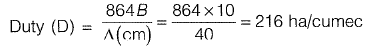Q. 8 Wheat crop requires 55 cm of water during 120 days of base period. The total rainfall during this period is 100 mm. Assume the irrigation efficiency to be 60%. The area (in ha) of the land which can be irrigated with a canal flow of 0.01 m3/s is
(a) 13.82
(b) 18.85
(c) 23.04
(d) 230.40     [2012 : 2 Marks]

Ans: (a)
Base period, 6 = 120 days
Δ = Net water required by the crop during base period
= Total water requirement - Rainfall
= 55 - 10 = 45 cm = 0.45 m
Now,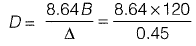⇒ D - 2304 ha/cumec
and Q= 0.01 m3/s
∴ Area = A = D x Q
= 2304 x 0.01 ha
= 23.04 ha
Efficiencyof irrigation = 60%
∴ Area which can be irrigated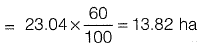Q. 9  The moisture holding capacity of the soil in a 100 hectare farm is 18 cm/m. The field is to be irrigated when 50 percent of the available moisture in the root zone is depleted. The irrigation water is to be supplied by a pump working for 10 hours a day, and water application efficiency is 75 percent. Details of crops planned for cultivation are as follows: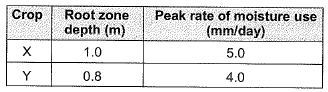The area of crop 'Y’ that can be irrigated when the available capacity of irrigation system is 40 litres/sec is
(a) 40 hectares
(b) 36 hectares
(c) 30 hectares
(d) 27 hectares     [2010 : 2 Marks]

Ans: (d)
Moisture holding capacity of soil for crop
Y = 18 x 0.8 = 14.4 cm
Allowable depletion of moisture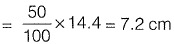Consumptive use of water for crop,
Y = 0.4 cm/day
∴ Frequency of irrigation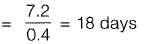Thus 7.2 cm depth of water is to be applied to the crop Y in the next 18 days.
Irrigation requirement =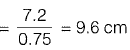Quantity of water required = Discharge through pump when pump works @ 10 hrs per day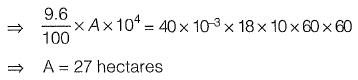Q. 10
The moisture holding capacity of the soil in a 100 hectare farm is 18 cm/m. The field is to be irrigated when 50 percent of the available moisture in the root zone is depleted. The irrigation water is to be supplied by a pump working for 10 hours a day, and water application efficiency is 75 per cent. Details of crops planned for cultivation are as follows:The capacity of irrigation system required to irrigate crop ‘X in 36 hectares is
(a) 83 litres/sec
(b) 67 litres/sec
(c) 57 litres/sec
(d) 53 litres/sec    [2010 : 2 Marks]

Ans: (b)
Moisture holding capacity of soil = 18 cm /m
Root zone depth of crop X = 1.0 m
Moisture holding capacity of soil for crop
X = 18 x 1 = 18cm
Allowable depletion of moisture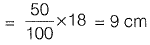Consumptive use of water for crop
X = 0.5 cm/day
∴ Frequency of irrigation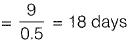Thus 9 cm depth of water is to be applied to the crop Xin the next 18 days.

Irrigation requirement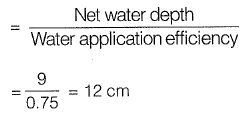Quantity of water required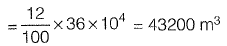∴ Discharge through pump (if pump works for 10 hrs per day)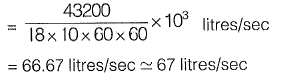Offer running on EduRev: Apply code STAYHOME200 to get INR 200 off on our premium plan EduRev Infinity!

## Irrigation Engineering

7 videos|15 docs|12 tests

,

,

,

,

,

,

,

,

,

,

,

,

,

,

,

,

,

,

,

,

,

;